GRE Subject Test: Physics : Astrophysics

Example Questions

Example Question #1 : Astrophysics

If the temperature of a blackbody doubles, what occurs to the wavelength of maximum emission?

It is halved.

It is quartered.

It doubles.

No change.

It is halved.

Explanation:

This is an application of Wein's Law that states the following: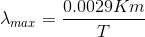, where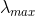is the wavelength of maximum emission of the object (measured in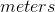), and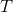is the temperature in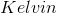. Since the wavelength and the temperature are inversely proportional, if we double the temperature, we must cut the wavelength by the same proportion. Therefore, the temperature must be halved.

Example Question #11 : Gre Subject Test: Physics

By what factor will the energy emitted each second off the surface of a blackbody change if the the temperature of the object is tripled?Explanation:

Stefan-Boltzmann's equation for a blackbody states: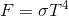, whereis the energy emitted each second,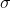is the Stefan-Boltzmann constant, andis the temperature of the blackbody. Therefore, if we triple the temperature, the energy will increase by a factor of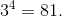Example Question #1 : Special Topics

Using the kinetic theory of gas, what is the fundamental property of a planet that dictates if it is able to retain an atmosphere?

Magnetic field strength

Temperature

Number of moons

Mass

Energy

Mass

Explanation:

The kinetic theory of gas states: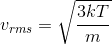. Whenever the velocity of the individual gas particles is larger than the escape speed of the planet,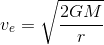, the gas particles will leak into space and deplete the atmosphere. The physical property that determines this is the mass of the planet.

All GRE Subject Test: Physics Resources